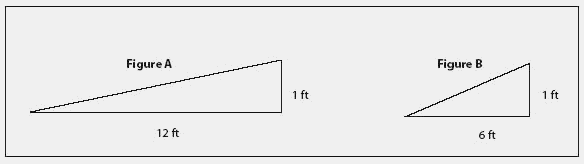Also found in: Dictionary, Thesaurus, Medical, Encyclopedia.The slope,or change in elevation,of land or improvements such as a pipe or a road.It is expressed as the ratio of inches (or feet) of rise or fall over a specified distance. It is similar to the concept of pitch in roofing.Figure A (above) has a gradient of 1:12 because the grade increases by 1 foot over a distance of 12 feet. Figure B has a gradient of 1:6.

The Complete Real Estate Encyclopedia by Denise L. Evans, JD & O. William Evans, JD. Copyright © 2007 by The McGraw-Hill Companies, Inc.
References in periodicals archive ?
Prince, "Generalized gradient vector flow external forces for active contours," Signal Processing, vol.
To calculate in parallel, the gradient vector is divided into groups which are executed in parallel.
(7) Repeat (4)-(6) steps grad_rep times; each time has an independent sample of [[DELTA].sub.i], and then finally acquire average gradient vector at [[theta].sub.i].
For both the outer and inner surface of the posterior sclera, a group of even sampled seed points were placed on the end circles around the equator and were traced by the directions of the gradient vector field derived from the harmonic scalar field.
The improved cost function integrates both modulus and phase components of the gradient vector and then combines them with SAD cost, leading to a superior accuracy.
An exact calculation of instantaneous gradient vector [nabla]J(n) is not possible because prior information on covariance matrix R and cross-correlation vector p is needed.
In continuous domain, the rate of change of a 2D image intensity function f(x, y) is given by the gradient vector, defined as
The novel algorithm is named "an optimal algorithm (OA)," because in the local frame we have derived the optimal parameter of a in the descent direction, which is a linear combination of the gradient vector and a supplemental vector.
The gradient vector of parameters respect to error function can be obtained by chain rule in which the optimum values of parameters are determined by iterative algorithms.
The presence of a heat flux vector will always be accompanied by a temperature gradient vector. The temperature gradient field is taken to be an indicator of conductive thermal resistance as, for a given heat flux, the greater the temperature gradient is, the larger the thermal resistance will be.
(7) Finally, the gradient vector is normalized and threshold to a particular level for display of edge map information.
Site: Follow: Share:
Open / Close